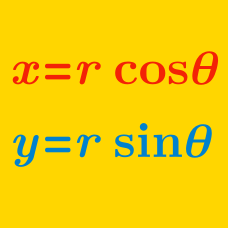Algebra

# Converting Cartesian Coordinates to Polar

The point $(5, 5\sqrt{3})$ in Cartesian coordinates can be expressed as $(r, \theta^{\circ})$ in polar coordinates, where $r$ is a positive real number and $0 \leq \theta \leq 180$. What is the value of $r+ \theta$?

The point $(-21\sqrt{3}, 21)$ in Cartesian coordinates can be expressed as $(r, \theta)$ in polar coordinates, where r is a positive real number and $0^{\circ} \leq \theta \leq 180^{\circ}$. If $\theta$ is measured in degrees, what is the value of $r+ \theta$?

If point $P$ is given in Cartesian coordinates as $P=(15, 15),$ what are the polar coordinates of $P?$

The point $(19, -19)$ in Cartesian coordinates can be expressed as $(q\sqrt{2}, \theta^{\circ})$ in polar coordinates, where $q$ is a positive real number and $0 \leq \theta \leq 360.$ What is the value of $q+ \theta$?

The point $(-19\sqrt{2}, 19\sqrt{2})$ in Cartesian coordinates can be expressed as $(r, \theta)$ in polar coordinates, where $r$ is a positive real number and $0^{\circ} \leq \theta \leq 180^{\circ}$. If $\theta$ is measured in degrees, what is the value of $r+\theta$?

×